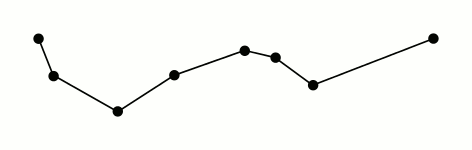# simplify_preserve_topology(...)

### simplify_preserve_topology(...)

Reduces the number of vertices in a line or polygon, preserving topology

Works with the following datatypes:

This function works with 2.1 endpoint(s). What does this mean? »

Similar to simplify(...), the simplify_preserve_topology(...) function reduces the number of vertices in a line or polygon using the Ramer–Douglas–Peucker algorithm. This is useful for approximation or for reducing the total size of a geospatial dataset.Unlike simplify(...), this function will not over-simplify geometries.

simplify_preserve_topology(...) takes two arguments:

• The geometry to be simplified
• The tolerance, in meters, used to simplify the segments in your geometry.

For example, to get a simplified version of the polygon for the City of Seattle from the Tiger LINE “Places” dataset: